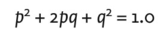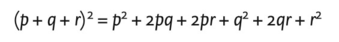# binomial expansion equation

## binomial expansion equation

an equation stating that (p + q)2 = 1.0, in which p and q are variables. The equation is used in population genetics (see POPULATION sense 2). Expansion of the term producesthe frequencies of the three genotypes expected in a population that has a gene with two alleles at frequencies of p and q respectively. The equation can be expanded to include additional alleles of a gene (see HARDY-WEINBERG LAW). For example, if a gene has three alleles with frequencies of p , q and r , the expected frequencies of the six possible genotypes would be:Collins Dictionary of Biology, 3rd ed. © W. G. Hale, V. A. Saunders, J. P. Margham 2005
Site: Follow: Share:
Open / Close